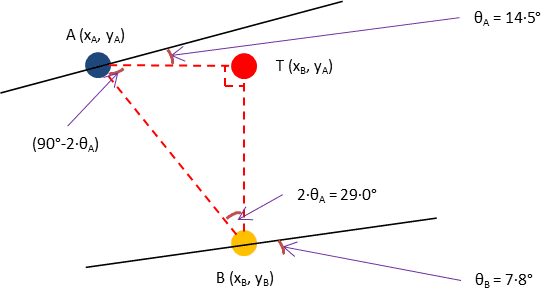# Translating points on the axes

• I
• RichardWattUK

#### RichardWattUK

TL;DR Summary
I need to translate points on coordinate axes as part of a calculation process
Summary: I need to translate points on coordinate axes as part of a calculation process

Hello everyone,

I've created the diagram below to try and explain what I am trying to do as part of an existing software app that's used to generate profiles and programs to drive a CNC machine to grind worm gears.

The worm profile is translated by rotating point "A" (which is on a linear profile) in the diagram below to point "B" (which is on a curved profile more like how it will be when ground by the machine), and this curved profile is later used for some other stages in the calculation process.The angles θA and θB represent the angle between the X axis and the outward normal vector from points A and B respectively.

There's an existing routine that uses the Newton-Raphson method to find a rotation angle β to perform this translation and is given the following values:
• Point A (xA, yA, θA)
• Projection angle (γA)
• Starting values
• Point B (xB, yB, θB)

This routine uses a Newton-Raphson loop to find a value of β that satisfies: C1 ⋅ cos β + C2 ⋅ sin β - C3 = 0 and doesn't always find a solution for all possible combinations of parameters provided by the user.

The values of C1, C2 and C3 are known before starting the iteration by calculating the following:

CA = cos αA
SA = sin αA
CG = cos γA
SG = sin γA
CT = -cos θA
ST = sin θA

C1 = SA ⋅ CG ⋅ CT
C2 = CA ⋅ CG ⋅ ST
C3 = CA ⋅ SG ⋅ CT

I've found an alternative method to calculate β by solving C1 ⋅ cos β + C2 ⋅ sin β = C3 based on the information in this Quora article.

Once I've got a value for φ from this, I use the following to work out the value of β:

φ = sin-1 (C1 / √(C12 + C22))
If |C3 / (C12 + C22)| Then
β = sin-1 (C3 / (C12 + C22)) - φ
Else
β = tan-1 (C2 / C1) - φ
End If
SB = sin β
CB = cos β

The coordinates of point B are then calculated from:

xB = β ⋅ Lead / 2π + xA
yB = yA ⋅cos β
θB = tan-1 ((-CA ⋅ CT) / (CA ⋅ CB ⋅ CG ⋅ ST - SA ⋅ SB ⋅ CG ⋅ -CT)) ⋅ (180 / π)

My question is: can anyone see a better way of doing this and/or any improvements that I can make to this method?

To improve your chances for a reply: Could you just summarize in a list, which values you know, and which you want to calculate? This would make things a lot easier to read (in combination with your picture).

•jedishrfu
I already know the following values:
• Point A (xA, yA, θA)